Next: 7.3 Technology B: Pseudomorphic HEMT Up: 7.2 The Bias Dependence Previous: 7.2.5 The Current Gain

## 7.2.6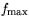and Related Quantities

Expressingas a function of small-signal equivalent elements, makes this quantity very sensitive to the approximation taken. Therefore, for circuit designis best described considering frequencywhere the stability factor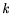= 1, and the gain MAG=MSG available at this frequency.is then determined from the transition MAG/MSG, and as both MAG and MSG have a defined slope as a function of frequency as given in Chapter 4,can be determined based on a number of data points.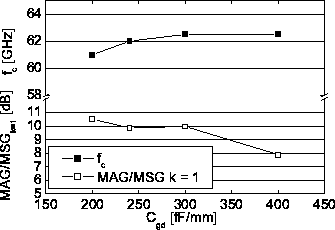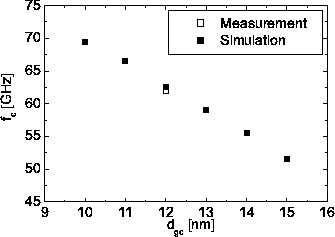Fig. 7.18 supplies information for a given bias, which gate width is feasible for power application at a given frequency. Fig. 7.18 shows the measured and modeled (compact modeling) dependence of MAG/MSG for k=1 varying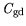in a small-signal model. All other parameters are kept constant. It can be seen, that for lowerthe gain MAG/MSG rises, while the frequencyslightly drops. The overall effects is a rise of. In order to understand the variations of this important relation due to further process variations, Fig. 7.19 shows the sensitivity analysis of the=1 point for a 4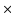40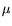m HEMT towards the gate-to-channel separation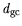simulated with MINIMOS-NT.rises significantly for decreasing gate-to-channel separation. All other device parameters are kept constant.Next: 7.3 Technology B: Pseudomorphic HEMT Up: 7.2 The Bias Dependence Previous: 7.2.5 The Current Gain
Quay
2001-12-21